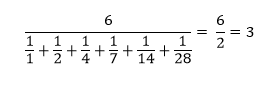# Check if the given number is Ore number or not in Python

Suppose we have a number n. We have to check whether n is an ore number or not. As we know an ore number is a number whose divisors have an integer harmonic value.

So, if the input is like 28, then the output will be True as there are six divisors of 28: [1, 2, 4, 7, 14, 28], soAs 3 is an integer so 28 is an ore number.

To solve this, we will follow these steps −

• Define a function get_all_div() . This will take n
• div := a new list
• for i in range 1 to integer part of (square root of n), do
• if n is divisible by i, then
• if quotient of (n / i) is i, then
• insert i at the end of div
• otherwise,
• insert i at the end of div
• insert quotient of (n / i) at the end of div
• return div
• Define a function get_harmonic_mean() . This will take n
• div := get_all_div(n)
• total := 0
• length := size of div
• for i in range 0 to length - 1, do
• total := total + (n / div[i])
• total := total / n
• return length / total
• From the main method do the following:
• mean := get_harmonic_mean(n)
• if mean is an integer, then
• return True
• return False

Let us see the following implementation to get better understanding −

## Example Code

Live Demo

def get_all_div(n):
div = []
for i in range(1, int(n**(0.5)) + 1):
if n % i == 0:
if n // i == i:
div.append(i)
else:
div.append(i)
div.append(n // i)
return div

def get_harmonic_mean(n):
div = get_all_div(n)

total = 0
length = len(div)

for i in range(0, length):
total += (n / div[i])

total /= n
return length / total

def solve(n):
mean = get_harmonic_mean(n)
if mean - int(mean) == 0:
return True
return False

n = 28
print(solve(n))

## Input

28

## Output

True